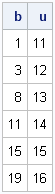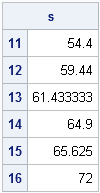Last week I showed how to use the UNIQUE-LOC technique to iterate over categories in a SAS/IML program. The observant reader might have noticed that the algorithm, although general, could be made more efficient if the data are sorted by categories.

### The UNIQUEBY Technique

Suppose that you want to compute a statistic for some variable for each unique level (category) of a categorical variable. To be concrete, assume you want to compute the mean height for students in the Sashelp.Class data, grouped by their age. The AGE variable defines the levels AGE=11, AGE=12, ..., and AGE=16. For this simple statistic, you could call PROC MEANS and specify AGE as a classification variable on the CLASS statement. But how can you compute the same quantities in SAS/IML software?

The UNIQUE-LOC technique is a simple and effective method for solving this problem. However, if you have a large quantity of data, it is more efficient to sort the data by the categorical variable. The following steps describe the UNIQUEBY technique for iterating over (sorted) levels of a categorical variable:

1. If the data are not sorted by the categorical variable, sort them.
2. Use the UNIQUEBY function to obtain the row numbers for the first observation in each level.
3. Allocate a vector to hold the statistics, one for each level.
4. Use a DO loop to iterate over the unique values.
5. For the ith level of the categorical variable, use the row numbers were computed by the UNIQUEBY function to find the observations in the level.
6. Extract the corresponding numerical values, and compute the statistic for those values.

This is similar to the general UNIQUE-LOC technique, except that you do not need to use the LOC function. Instead, you can use the results of the UNIQUEBY function to find the observations in each level.

The following SAS/IML statements show the first step of the algorithm: sorting the data by a numerical categorical variable.
```proc iml; use sashelp.class; read all var {age height} into m; close; call sort(m, 1); /* 1. Sort data by first column */ C = m[,1]; /* finished with m: extract sorted categories */ x = m[,2]; /* and sorted data */```

In the preceding code, I've used the SORT subroutine in SAS/IML to sort numerical data, but if the data are sorted prior to calling PROC IML, you can read the data directly into C and x. Of course, if the categorical variable is character, you cannot form the matrix m because a matrix must be either all numerical or all character. In that case, you can use PROC sort to pre-sort the data, or you can call the SORTNDX subroutine in SAS/IML to order x according to the values in C.

Regardless of how you accomplish the sorting, the subsequent steps assume that C contains the sorted categories, and that x contains the sorted data. The following SAS/IML statements compute the row numbers that indicate the beginning of each category:

```/* 2. Obtain row numbers for the first observation in each level. */ b = uniqueby(C, 1); /* b[i] = beginning of i_th category */ u = C[b]; /* get unique values (if needed) */ print b u;```The first category (AGE=11) starts on the row 1, the second category (AGE=12) starts on row 3, and so forth. Consequently, the observations for the first category are from b to b-1. Similarly, the observations for the second category are from b to b-1. In general, the observations for the ith category are b[i] to b[i+1]-1. Well, almost. This formula doesn't work for the last category. However, if we add the value nrow(C)+1 to the end of the b vector, then the formula can be used for all categories. The following SAS/IML statements implement this trick and the rest of the the algorithm:

```s = j(nrow(b),1); /* 3. Allocate vector to hold results */ b = b // (nrow(C)+1); /* trick: append (n+1) to end of b */ do i = 1 to nrow(b)-1; /* 4. For each level... */ idx = b[i]:(b[i+1]-1); /* 5. Find observations in level */ s[i] = mean(x[idx]); /* 6. Compute statistic on those values */ end; lbl = putn(u, "Best4."); /* convert numeric values to character */ print s[rowname=lbl];```Success! By using the trick prior to the DO loop, the algorithm can treat all categories the same. This approach is usually more efficient than using an IF-THEN/ELSE statement inside of a loop to handle special looping situations.

The UNIQUEBY technique offers an alternative to the useful UNIQUE-LOC technique for iterating over levels of a categorical variable. It is more efficient than the UNIQUE-LOC technique because it does not need to use the LOC function to find the observations in each category. Instead, the UNIQUEBY subroutine finds the row numbers at the beginning of each category, and you can use those row numbers to iterate over the categories.

Share